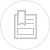# fundamental concepts on the oxidation-reduction chemical reaction

#### the oxidising-reduction reaction and the redox couple

Each oxidation-reduction reaction can be described using half-equations applicable to the two Redox couples involved such that the electrons released by reducer 2 are transferred to oxidant 1.

The number of electrons captured by Ox1 must be equal to the number of electrons released by Red2 so that the global reaction is obtained by combining, term by term, the two partial equations each multiplied by the number of electrons involved in the other. For example, ferrous ion oxidation by permanganate in an acidic aqueous solution is written:

#### foreseen oxidation-reduction reactions

The equilibrium direction with shift depending the relative force of the Ox1/Red1 and Ox2/Red2 redox couples. The force of each redox couple is provided by the standard potential written E° and established in relation to the reference couple: H3O+/H2 at 0 pH and temperature T.

Thus, for the reaction to take place to the right, we need K > 1, i.e. oxidiser 1 and reducer 2 must respectively be stronger than oxidiser 2 and reducer 1 which means a higher standard potential for couple 1 than for couple 2:. E1° > E2°.

The normal standard potential values (T = 298 K) for certain pairs of compounds found in water are given in table 23 which is complementary to table 15 (see section basic priniciples - definitions).

#### oxidation degree or number

If it is an easy matter to identify the most oxidised species in metal element pairs such as Fe3+/Fe2+, this identification becomes more difficult in the case of various more complex compounds of the same element such as in the chloride ion (Cℓ), chlorine (Cℓ2), hypochlorite ion (CℓO), chlorite ion (CℓO2), chlo­rate ion (CℓO3) and perchlorate ion (CℓO4) series. Here, we will use the degree of oxidation or the oxidation number (written ON) for a given atom which matches the number of electrons involved to form the molecular or ionic compound from a pure substance, assuming that all the element bonds in the compound are ionic. The oxidation number will be positive when the conversion is an oxidation and negative when a reduction is required. It is shown as a Roman numeral in order to distinguish it from the ionic charge. It is established by applying the following rules:

• all elements in the pure state have the oxidation number 0;
• in a molecule, the sum of the oxidation numbers of the constituent elements, weighted by their number, is equal to 0;
• in an ionic species, the sum of the oxidation numbers of the constituent elements, weighted by their number, is equal to the ion’s charge;
• hydrogen’s oxidation number is +1 (except its hydride where NO(H) = – I);
• oxygen’s oxidation number is – II (except in the case of peroxides where NO(O) = – I).

In the chlorine compound series, the chlorine oxidation number will then be – I for Cℓ, 0 for Cℓ2, + I for CℓO, + III for CℓO2, + V for CℓO3 and + VII for CℓO4.

The higher the oxidation number, the more the element is oxidised.

Calculation of oxidation degrees is particularly indicated when the oxidation-reduction partial equations are not known as in the case of most organic compounds. In effect, this calculation enables us to establish the global equation according to the principles whereby the oxidation number and the material are retained. If we revert to the example of the ferrous ion reaction with permanganate in an acid medium:

#### variation of the oxidation reduction potential according to the pH

Some partial oxidation reduction reactions involve water molecules H2O, protons H+ and hydroxyl ions OH for maintaining electrical charges in equilibrium. In this case, the oxidation reduction potential will depend on the pH. For a redox pair characterised by a partial equation of the type: